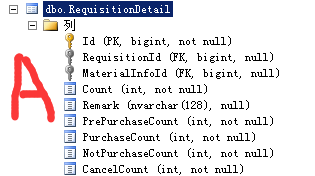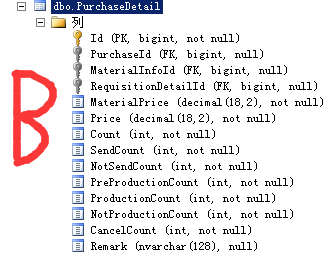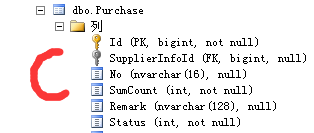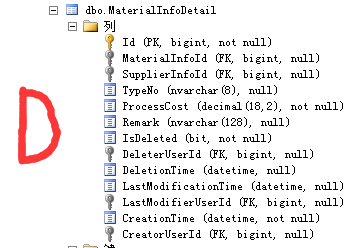ef linq 多表查询条件问题 5CA 表是要查询的表 B表和C表是父子关系

A表是申购单明细 B表是采购单明细 C表是采购单
B表和A表有字段关联

3个回答

var tempRes = form b in B,c in C where b.PurchaseId = c.id and (c.Status==0 or c.Status == 1)
select b.count,b.RequisitionDetailId;

from a in A where a.Count !=(from t in tempRes where t. RequisitionDetailId = a.id select count).Sum()
select a;var t = from a1 in A
join a2 in (
from a in A
join b in B on a.ID equals b.RequisitionDetailId
join c in C on b.PurchaseID equals c.ID
where c.Status == 0 || c.Status == 1
group a by a.ID into temp
select new { aID = temp.Key, bcount = temp.Count() })
on a1.ID equals a2.aID where a1.Count != a2.bcount select a1;var tempRes = form b in B,c in C where b.PurchaseId = c.id and (c.Status==0 or c.Status == 1)
select b.count,b.RequisitionDetailId;

from a in A where a.Count !=(from t in tempRes where t. RequisitionDetailId = a.id select count(t.RequisitionDetailId))
select a;